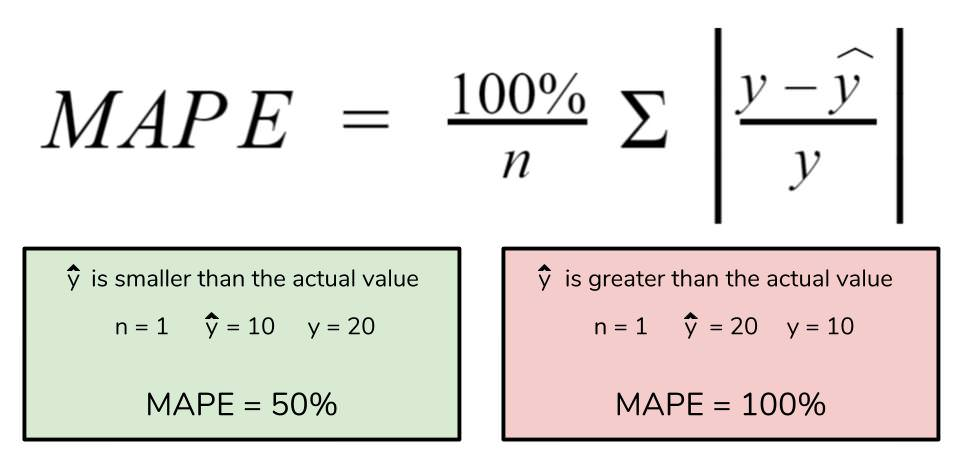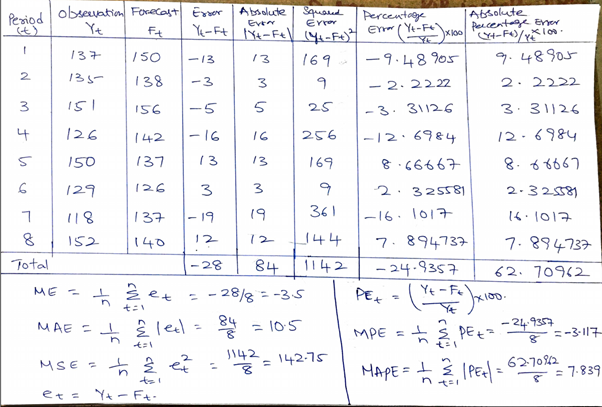# 23 Forecast ErrorIf, generically, for the period $$t$$ a prediction is made with the information available at $$t-1$$, which we denote by $$hat{y}_{t|t-1}$$, for the period $$t$$ we can make a comparison of this value with the one we actually observe ($$y_t$$). The difference between both values will be the prediction error one period forward and will be given by:

$e_{t | t-1}=y_t-\hat y_{t| t-1}$

To quantify the overall prediction errors, the following statistics are used: the Root Mean Squared Error (RQE) and the Mean Absolute Error (MAE).

In the case that $$T$$ observations are available and forecasts have been made from the observation $$2$$, RECM and EAM are given by

$RECM=\sqrt{\frac{\sum_{t=2}^T (y_t-\hat y_{t/t-1})^2}{T-1}}$

$EAM=\dfrac{1}{T-1}\sum_{t=2}^T \left(y_t-\hat y_{t/t-1}\right)^2$

We can also use the MAPE, Mean Absolute Percentage Error.

$MAPE=\dfrac{1}{T-1}\sum_{t=2}^T \left( \dfrac{ \hat y_{t/t-1}}{y_t} \right)$